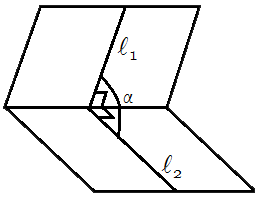Study of mathematics online.
Study math with us and make sure that "Mathematics is easy!"

# Angle between two planesDefinition.
The angle between planes is equal to a angle between their normal vectors.

Definition.
The angle between planes is equal to a angle between lines l1 and l2, which lie on planes and which is perpendicular to lines of planes crossing.

## Angle between two planes formulas

If A1x + B1y + C1z + D1 = 0 and A2x + B2y + C2z + D2 = 0 are a plane equations, then angle between planes can be found using the following formula

 cos α = |A1·A2 + B1·B2 + C1·C2| √A12 + B12 + C12√A22 + B22 + C22

## Examples of tasks with angle between two planes

Example 1.
To find an Angle between planes 2x + 4y - 4z - 6 = 0 and 4x + 3y + 9 = 0.

Solution. Let's use the formula:

 cos α = |2·4 + 4·3 + (-4)·0| = |8 + 12| = 20 = 2 √22 + 42 + (-4)2√42 + 32 + 02 √36√25 30 3
 Answer: the cosine of the angle between the planes is cos α = 2 . 3